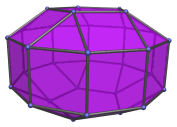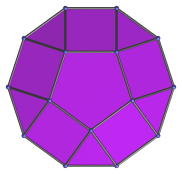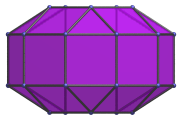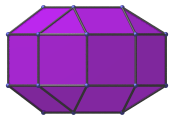# The Elongated Pentagonal Orthobicupola

The elongated pentagonal orthobicupola is the 38th Johnson solid (J38). It has 30 vertices, 60 edges, and 32 faces (10 equilateral triangles, 20 squares, 2 pentagons).The elongated pentagonal orthobicupola can be constructed by attaching two pentagonal cupolae to a decagonal prism, or equivalently, inserting a decagonal prism between the two halves of the pentagonal orthobicupola (J30). The ortho- in the name refers to how the top and bottom pentagons are aligned with each other. If they are rotated with respect to each other instead, the elongated pentagonal gyrobicupola (J39) is produced instead.

## Projections

Here are some views of the elongated pentagonal orthobicupola from various angles:

Projection DescriptionTop view.Front view.Side view.

## Coordinates

The Cartesian coordinates of the elongated pentagonal orthobicupola with edge length 2 are:

• (0, √((10+2√5)/5), ±(1+2√((3−φ)/5)))
• (±φ, √((5−√5)/10), ±(1+2√((3−φ)/5)))
• (±1, −√((5+2√5)/5), ±(1+2√((3−φ)/5)))
• (±2φ, 0, ±1)
• (±1, ±√(3+4φ), ±1)
• (±φ2, ±√(2+φ), ±1)

where φ=(1+√5)/2 is the Golden Ratio.

Last updated 17 May 2018.